# Acoustics and Vibration Animations

Daniel A. Russell, Graduate Program in Acoustics, The Pennsylvania State UniversityThis work by Dan Russell is licensed under a Creative Commons Attribution-NonCommercial-NoDerivatives 4.0 International License.

# Vibrational Modes of a Circular Membrane

The content of this page was originally posted on January 21, 1998. Animations were updated on August 29, 2018.

NOTE: in the following descriptions of the mode shapes of a circular membrane, the nomenclature for labelling the modes is (d,c) where d is the number of nodal diameters and c is the number of nodal circles. A node is a point (or line) on a structure that does not move while the rest of the structure is vibrating. On the animations below, the nodal diameters and circles show up as white regions that don't oscillate, while the red and blue regions indicate positive and negative displacements.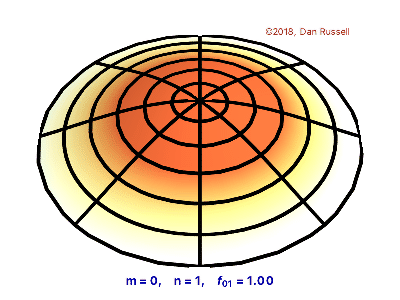### The (0,1) Mode

The animation at left shows the fundamental mode shape for a vibrating circular membrane. The mode number is designated as (0,1) since there are no nodal diameters, but one circular node (the outside edge). The (0,1) mode of a drum, such as a tympani, is excited for impacts at any location on the drumhead (membrane). When vibrating in this mode the membrane acts much like a monopole source, which radiates sound very effectively. Since it radiates sound so well when vibrating in this manner, the membrane quickly transfers its vibrational energy into radiated sound energy and the vibration dies away. The short duration (fraction of a second) of the (0,1) mode means that this mode does not contribute greatly to the musical tone quality of a drum. In fact, when struck at the center a tympani, or other large drum, produces a "thump" which decays quickly and with no definite pitch.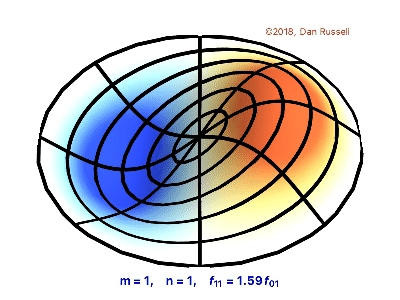### The (1,1) Mode

The next mode is the (1,1) mode with one nodal diameter and one circlular node (the outside edge). The exact location of the nodal diameter depends on the homogeneity of the membrane and the initial conditions when the vibration starts. The frequency of the (1,1) mode is 1.593 times the frequency of the (0,1) mode. When vibrating in the (1,1) mode a circular membrane acts much like a dipole source; instead of pushing air away from the membrane like the (0,1) mode does, in the (1,1) mode one half of the membrane pushes air up while the other half sucks air down resulting in air being pushed back and forth from side to side. As a result, the (1,1) mode radiates sound less effectively than the (0,1) mode which means that it does not transfer its vibrational energy into radiated sound energy as quickly as the (0,1) mode and therfore, the (1,1) mode takes longer to decay. Because the (1,1) mode "rings" for a while, it contributes to the musical sound or pitch of a drum. When a tympani, or other large drum, is struck somewhere between the center and outer edge, the sound has a definite pitch which lingers for a several seconds.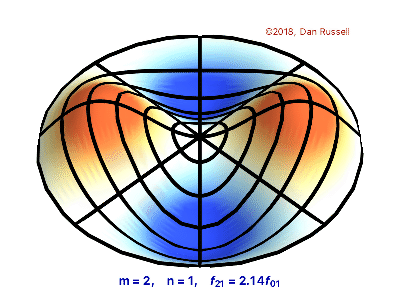### The (2,1) Mode

The third mode of a circular membrane is the (2,1) mode which has two nodal diameters (at right angles to each other) and one nodal circle (the outside edge). The exact locations of the nodal diameters depend on the homogeneity of the membrane and the initial conditions when the vibration starts. The frequency of the (2,1) mode is 2.135 times the frequency of the (0,1) mode. When vibrating in the (2,1) mode a circular membrane acts much like a quadrupole source which is worse at radiating sound than the (1,1) dipole mode and much less effective at radiating sound than the (0,1) monopole mode. This means that the (2,1) transfers its vibrational energy into radiated sound energy much more slowly than the (1,1) and (0,1) modes and therefore takes longer to decay, and contributes to the musical pitch of a drum. In fact, the modes which most significantly determine the tone quality of a tymani drum are the (1,1), (2,1), (3,1), (4,1), and (5,1) modes.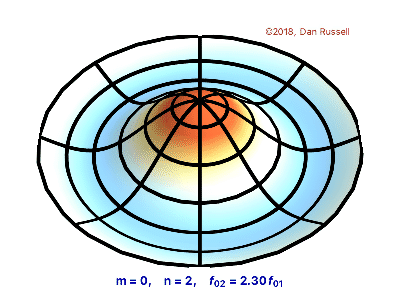### The (0,2) Mode

The (0,2) mode, shown at right does not have any diameter nodes, but has two circular nodes - one at the outside edge and one at a distance of 0.436 a from the center of the membrane (with a being the radius of the circular membrane). The frequency of the (0,2) mode is 2.295 times the frequency of the (0,1) mode. The sound radiation characteristics of the (0,2) mode are more complicated than the first three modes -- it appears to be a mix between a monopole and a dipole. Its decay time is longer than the (0,1) mode, but shorter than the (1,1) mode. As a result, it contributes to the "thump" sound when a drum is hit at the center, but does not contribute much to the musical pitch of a drum when hit off center.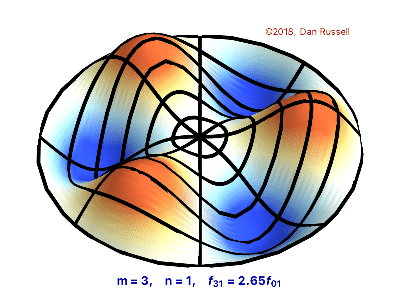### The (3,1) Mode

The fifth mode of a circular membrane is the (3,1) mode which has three nodal diameters and one nodal circle (the outside edge). Like the (1,1) and (2,1) modes, this pattern is a poor radiator or sound and therefore takes longer to decay, and contributes to the musical pitch of a drum.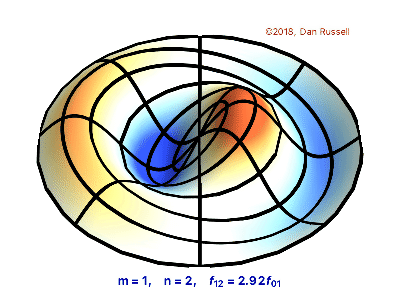### The (1,2) Mode

The (1,2) mode has one nodal diameter and two nodal circles. The frequency of the (1,2) mode is 2.917 times the frequency of the (0,1) mode. As you might expect after looking at the first several modes of the circular membrane, the (1,2) mode does not radiate sound very effectively. It has somewhat of a quadrupole type behavior. Thus, the (1,2) mode takes a relatively long time to decay. However, this mode doesn't seem to play a dominant role in the musical tone quality of a drum.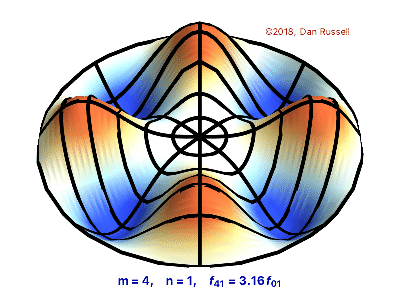### The (4,1) Mode

The seventh mode of a circular membrane is the (4,1) mode which has four nodal diameters and one nodal circle (the outside edge). Like the (1,1), (2,1), (3,1) modes, this pattern is a poor radiator or sound and therefore takes longer to decay, and contributes to the musical pitch of a drum.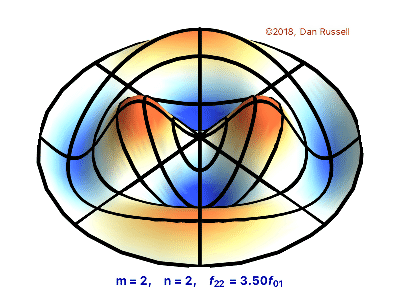### The (2,2) Mode

The eighth mode of a circular membrane is the (2,2) mode which has two nodal diameters and two nodal circles.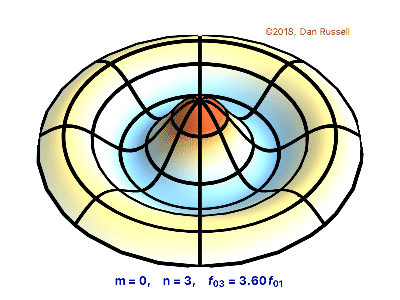### The (0,3) Mode

The (0,3) mode has three circular nodes, but no diameter nodes. The frequency of the (0,3) mode is 3.598 times the frequency of the (0,1) mode. The sound radiation characteristics of the (0,3) mode rather complicated. However, this mode is excited when the membrane is struck at the center, and it dies away fairly quickly. As a result, it contributes to the "thump" sound when a drum is hit at the center, but does not contribute much to the musical pitch of a drum when hit off center.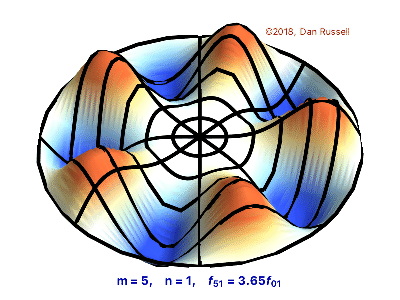### The (5,1) Mode

The tenth mode of a circular membrane is the (5,1) mode which has five nodal diameters and one nodal circles at the fixed outer rim.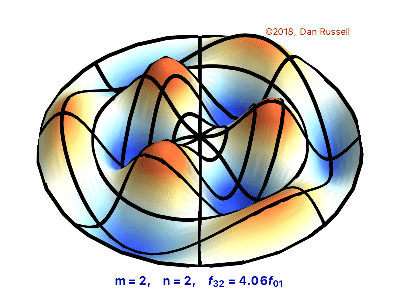### The (3,2) Mode

The eleventh mode of a circular membrane is the (3,2) mode which has three nodal diameters and two nodal circles.

NOTE => the mode designation underneath the mode shape is incorrectly labeled (m=2) instead of (m=3). I have lost the code used to generate these animations, and have not had the time to re-create it. Sorry for the inconvenience.